# Class 9 Maths Problems for Number System

Notes Ncert Solutions Assignments Videos Revision sheet

Here we are trying to give the Class 9 Maths Problems for Number System. It tests the basic concepts and at the same makes the student comfortable with the questions

Question 1
Find the value of each of the Following
a) 21/4.21/5
b) 16-3/4
c)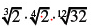d) 15√6 + √216
e) 115/2 / 113/2
f) 53/2  73/2
g) √162/√2
h) (8+√3)/(8-√3)
Question 2
Simplify the below expression
a)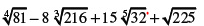b)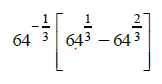c)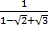d)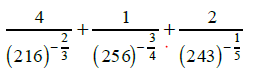Question 3
Find the value of a and b such that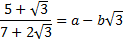Question 4
If x = 1+√3
and
y=1/x
Find the value of
i) x2 + y2 ii) x2 - y2 iii) (x+y)2 iv) x-y Question 5
Write the following in decimal form and say what kind of decimal expansion each has:
(i) 19/100
(ii) 1/3
(iii) 11/12
(iv) 1/13
(v) 2/17
(vi) 111/400

link to this page by copying the following text